# How does reversible =>no entropy change in the universe?

## Homework Statement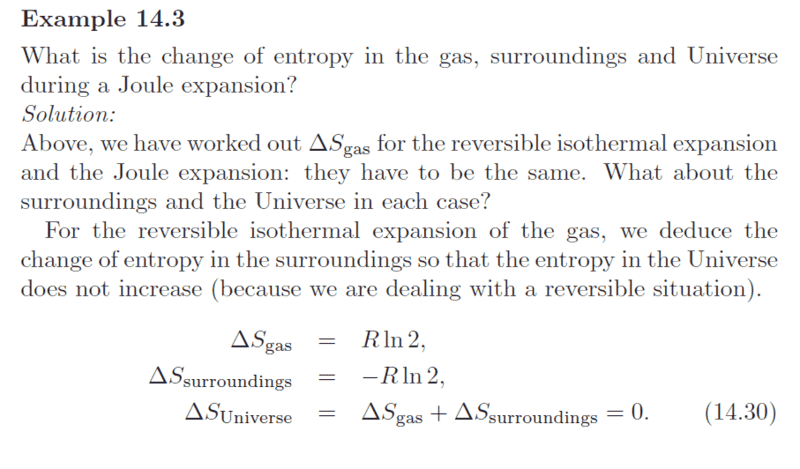2.Relevant equations[/B]

## The Attempt at a Solution

How does a reversible process in the universe imply the entropy doesn't increase? I understand that the change of entropy in a closed reversible cycle is 0 in the system, but I don't get why a not closed reversible process in a system can imply zero change in entropy in the universe.

#### Attachments

mfb
Mentor
If the overall entropy increases, what would the entropy do in the time-reversed process? Is that possible?

•Clara Chung
Chestermiller
Mentor
By a Joule expansion, do you mean that, initially you have an insulated volume divided in half, with gas in one chamber and vacuum in the other. You remove the barrier between the chambers and allow the system to re-equilibrate?

Chestermiller
Mentor

## Homework Statement

View attachment 236661

2.Relevant equations[/B]

## The Attempt at a Solution

How does a reversible process in the universe imply the entropy doesn't increase? I understand that the change of entropy in a closed reversible cycle is 0 in the system, but I don't get why a not closed reversible process in a system can imply zero change in entropy in the universe.
Clara,
You are confused over these results because they are incorrect, at least for the surroundings and for the universe. After you read my PF insights article on how to determine the entropy change for an irreversible process, we will have a basis for discussing why they are incorrect, and what the correct answers are.

George Jones
Staff Emeritus
Gold Member
Clara,
You are confused over these results because they are incorrect, at least for the surroundings and for the universe. After you read my PF insights article on how to determine the entropy change for an irreversible process, we will have a basis for discussing why they are incorrect, and what the correct answers are.

But this thread is not about an irreversible processs, it is about a reversible process, so Clara has not included the complete example. In the part of the example given, equation (14.30) and the equation preceding it are for a reversible isothermal expansion. In the part of the example not included, expressions are given for an irreversible Joule expansion.

Chestermiller
Mentor
But this thread is not about an irreversible processs, it is about a reversible process, so Clara has not included the complete example. In the part of the example given, equation (14.30) and the equation preceding it are for a reversible isothermal expansion. In the part of the example not included, expressions are given for an irreversible Joule expansion.
I have no idea what you mean. Joule expansion is an irreversible (spontaneous) process.

George Jones
Staff Emeritus
Gold Member
I have no idea what you mean. Joule expansion is an irreversible (spontaneous) process.
For completeness, I give below the complete example and the section from which the example is taken. In this particular thread, Clara is asking only about the solid line process of Fig. 14.5.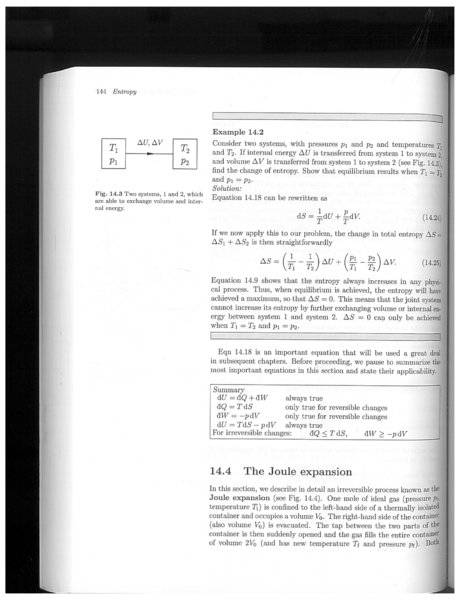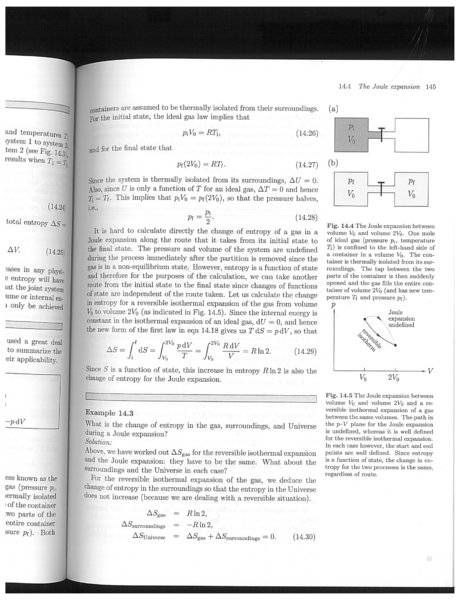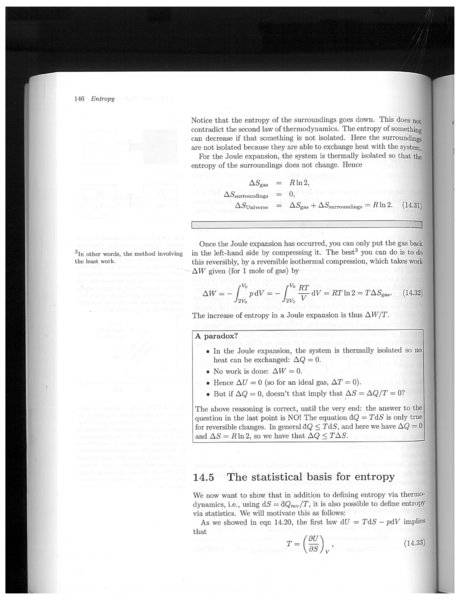#### Attachments

•Clara Chung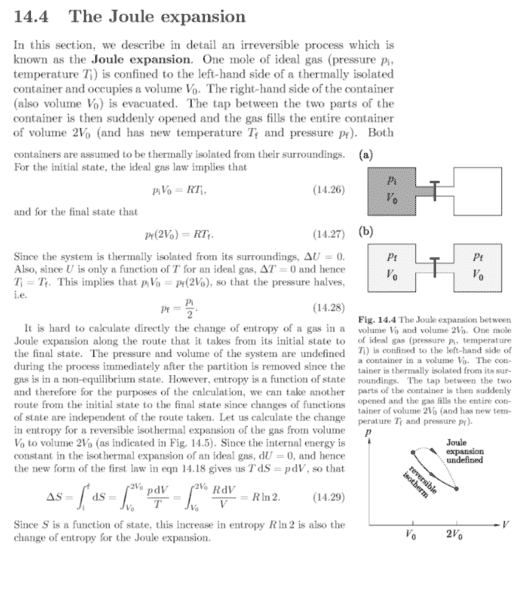I am sorry for not posting the whole section. This is the "above" section and I think the problem is discussing about the devised reversible process from two equilibrium states...(not the joule expansion.)
If the process is irreversible, the entropy change of the gas is still the same as the reversible process. However, in the universe the change of entropy is an upper bound of the integral of q'(t)/T, so the change of entropy must be larger than 0.

#### Attachments

Chestermiller
Mentor
View attachment 236693
I am sorry for not posting the whole section. This is the "above" section and I think the problem is discussing about the devised reversible process from two equilibrium states...(not the joule expansion.)
You are correct that it is discussing the devised reversible process. However, the devised reversible process has the same two end states as the irreversible Joule expansion. And, as I said in Step 2 of my Insights article, you need to totally forget about the actual process path when determining the change in entropy between the end states. It is no longer of consequence. The only thing that matters is the two end states.
If the process is irreversible, the entropy change of the gas is still the same as the reversible process.
Yes.
However, in the universe the change of entropy is an upper bound of the integral of q'(t)/T, so the change of entropy must be larger than 0.
We can determine the change in entropy of the universe and the change in entropy of the surroundings in a precise manner for the Joule expansion. Here's how. We now focus on the surroundings and treat it as a second separate "system" that can experience a possible change during the Joule expansion that occurred in the primary system. Treading the surroundings as a second system, please describe the change in the thermodynamic state of the surroundings between its initial and final states, when the gas in the closed insulated primary system experiences an irreversible Joule expansion.

Last edited:
•Clara Chung
We can determine the change in entropy of the universe and the change in entropy of the surroundings in a precise manner for the Joule expansion. Here's how. We now focus on the surroundings and treat it as a second "system" that can experience a possible change during the Joule expansion that occurred in the primary system. Treading the surroundings as a second system, please describe the change in the thermodynamic state of the surroundings between its initial and final states, when the gas in the closed insulated primary system experiences an irreversible Joule expansion.
There are no heat change and no change in internal energy of the second system. The original volume of the second system is V0, the final volume of it is Vf=V0 + V.
So, the initial pressure is P0 and final pressure Pf, the final pressure is given by the ideal gas law.
Pf = P0 * (V0 / V0 + V)
Now I forget about the irreversible and define a reversible process from the initial state to the final state which the macroscopic properties are well defined in the process. The step is an isothermal expansion from V0 to Vf, the entropy change in this reversible process will be nRln(Vf/V0). Also, after the isothermal expansion, the pressure matches the pressure of the final state in the irreversible process.
And the change of entropy of the universe will be the change in entropy of the second system plus the change in entropy of the first system......
?

Chestermiller
Mentor
There are no heat change and no change in internal energy of the second system. The original volume of the second system is V0, the final volume of it is Vf=V0 + V.
No. There is no change in the surroundings (this second separate system) whatsoever. It doesn't even know that anything happened in the rigid, insulated container. So, at the end of the irreversible change in the container where the Joule expansion took place, the final state of the surroundings is exactly the same as it was in its initial state. What does this tell you about the entropy change for the surroundings?

•Clara Chung
No. There is no change in the surroundings (this second separate system) whatsoever. It doesn't even know that anything happened in the rigid, insulated container. So, at the end of the irreversible change in the container where the Joule expansion took place, the final state of the surroundings is exactly the same as it was in its initial state. What does this tell you about the entropy change for the surroundings?
Ah...right... That will be equivalent with no process have ever happened, dQrev will be zero, so the entropy change of the surroundings is zero.

Chestermiller
Mentor
Ah...right... That will be equivalent with no process have ever happened, dQrev will be zero, so the entropy change of the surroundings is zero.
Right. So, now, what is the entropy change of the universe (system + surroundings)?

Chestermiller
Mentor
So what you see here is that, in determining the entropy change of a system, you can separate various parts of the system, and devise separate reversible paths for each of them. And, considering the system and surroundings as. part of a larger overall isolated system, you can separate the system from the surroundings, and devise separate reversible paths for each of them.

•Clara Chung
So what you see here is that, in determining the entropy change of a system, you can separate various parts of the system, and devise separate reversible paths for each of them. And, considering the system and surroundings as. part of a larger overall isolated system, you can separate the system from the surroundings, and devise separate reversible paths for each of them.
Thank you. The entropy change in the universe will be the entropy change in the primary system. I think I got the recipe too. Thank you

Chestermiller
Mentor
Thank you. The entropy change in the universe will be the entropy change in the primary system. I think I got the recipe too. Thank you
You can also now see how the answer in your book for this problem is incorrect. You have shown that, as expected, the increase in entropy of the universe for the irreversible Joule expansion is greater than zero.

Now what you need to do is get some practice applying this methodology (to lock it in). Maybe an interesting practice problem might be the one we discussed in the other thread, which also features an initial temperature difference between the two chambers. If you decide to go ahead with this, I will specify the problem statement in a precise manner.

George Jones
Staff Emeritus
Gold Member
You can also now see how the answer in your book for this problem is incorrect. You have shown that, as expected, the increase in entropy of the universe for the irreversible Joule expansion is greater than zero.

Maybe I am missing something, but how is this different from the answer given in the example from the book? The book's answer also gives that the increase in entropy of the universe for the irreversible Joule expansion is greater than zero.

Chestermiller
Mentor
Maybe I am missing something, but how is this different from the answer given in the example from the book? The book's answer also gives that the increase in entropy of the universe for the irreversible Joule expansion is greater than zero.
That's not what the image in post #1 seems to show. What am I missing?

George Jones
Staff Emeritus
Gold Member
That's not what the image in post #1 seems to show. What am I missing?

The image in post #1 is a subset of the images that I gave in post #8. The example starts on the page 145 and continues on to page 146. The three equations labeled (14.30) at the bottom of page 145 are for a reversible isothermal process. The three equations (14.31) at the top of page 146 are for the Joule expansion.